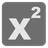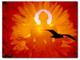Simple
English UK

Quadratic Equation may be expressed as a product

`a(x-x1)(x-x2) = 0,`
where x1 and x2 are the solutions of equation, so called roots. After opening the brackets you receive well known form
`a*x**2 + b*x + c = 0.`
This is what you should do in this mission. You have input list with integers - a, x1 [, x2]. If it has length 2, it means, x1 == x2: equation has two equal roots (one distinct root). Your function should return quadratic equation as a string. Pay attention to specific cases. Use Quadratic Formula Calculator for recheck. Good luck!

Input: List with 2-3 integers.

Output: String.

Examples:

```assert quadr_equation([2, 4, 6]) == "2*x**2 - 20*x + 48 = 0"
assert quadr_equation([-2, 4, 6]) == "-2*x**2 + 20*x - 48 = 0"
assert quadr_equation([2, 4, -4]) == "2*x**2 - 32 = 0"
assert quadr_equation([2, 4, 0]) == "2*x**2 - 8*x = 0"
```

How it’s used: Practice of automatic representing human-friendly view of equations.

Preconditions:

• a != 0; abs(a) == 1 -> '[-]x**2'; a != abs(1) - > '[-]a*x**2'
• exactly one whitespace around signs: [-]a*x**2 sign b*x sign c = 0
• abs(b) == 1 -> [-]a*x**2 sign x sign c = 0
• keep correct view and spacing when x1=x2=0, x1 or x2 = 0, x1=-x237
Settings
Code:
Other:
Invalid hot key. Each hot key should be unique and valid
Hot keys:
•  to Run Code: to Check Solution: to Stop:
CheckiO Extensions

CheckiO Extensions allow you to use local files to solve missions. More info in a blog post.

In order to install CheckiO client you'll need installed Python (version at least 3.8)

Install CheckiO Client first:

`pip3 install checkio_client`

`checkio --domain=py config --key=`

Sync solutions into your local folder

`checkio sync`

(in beta testing) Launch local server so your browser can use it and sync solution between local file end extension on the fly. (doesn't work for safari)

`checkio serv -d`

Alternatevly, you can install Chrome extension or FF addon

`checkio install-plugin`
`checkio install-plugin --ff`
`checkio install-plugin --chromium`

Read more here about other functionality that the checkio client provides. Feel free to submit an issue in case of any difficulties.